IntroductionIntroduction# Circumference and Area of Circles

INTRO
Remembering all the different parts of a circle and what they represent can sometimes feel a little out of reach. That’s why we like to think about them in terms of our favorite type of circle...pizza!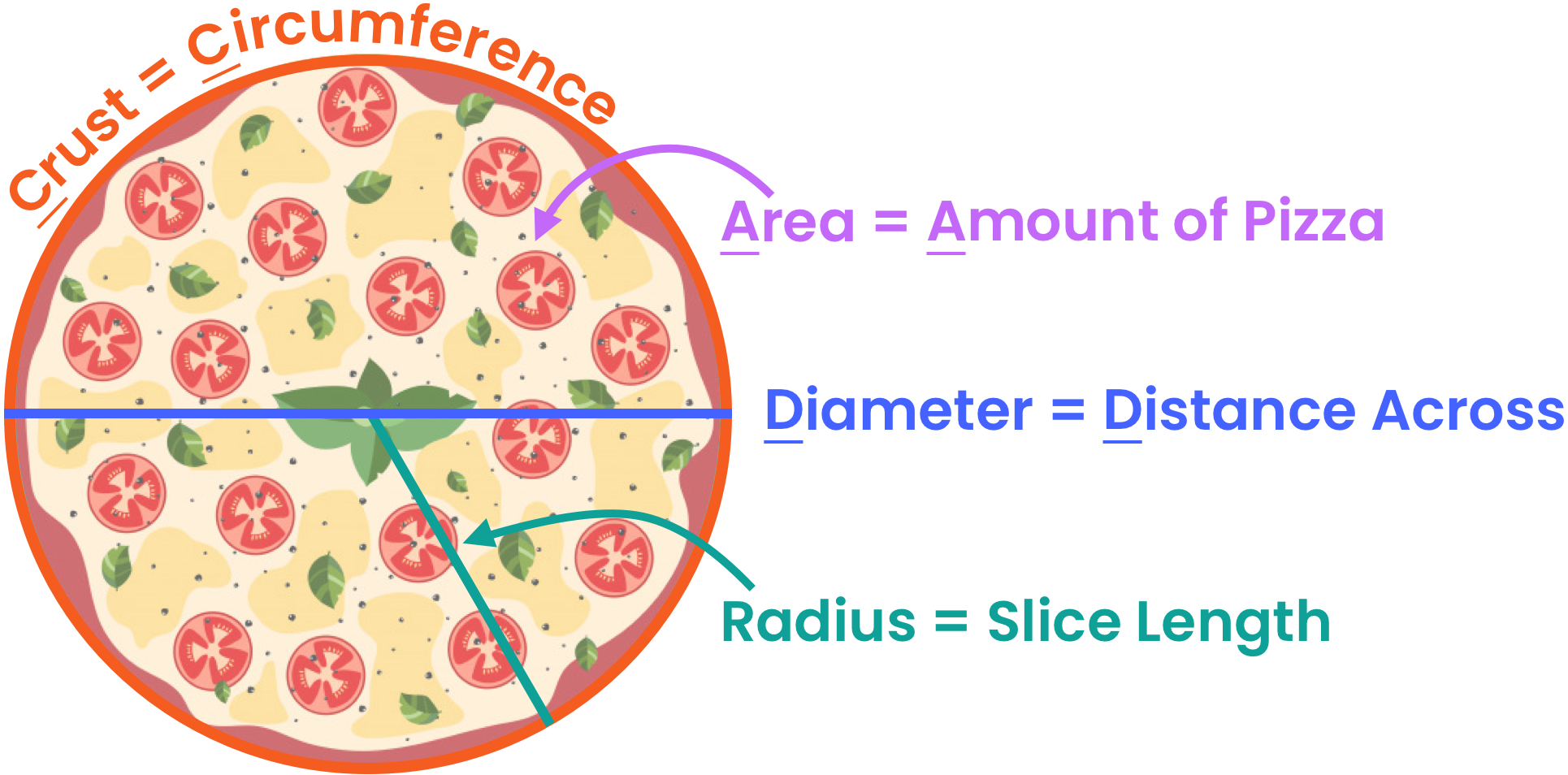These are different formulas we can use to find these different values:
 Circle Part Pizza Formula Circumference Crust Area Amount of Pizza Diameter Distance Across Radius Slice Length
Check out our
Calculator
or explore our
Lesson
and
Practice

CALCULATOR

## Circumference & Area of Circles Calculator

#### Step 1. Identify the unknown.

What are you trying to solve for?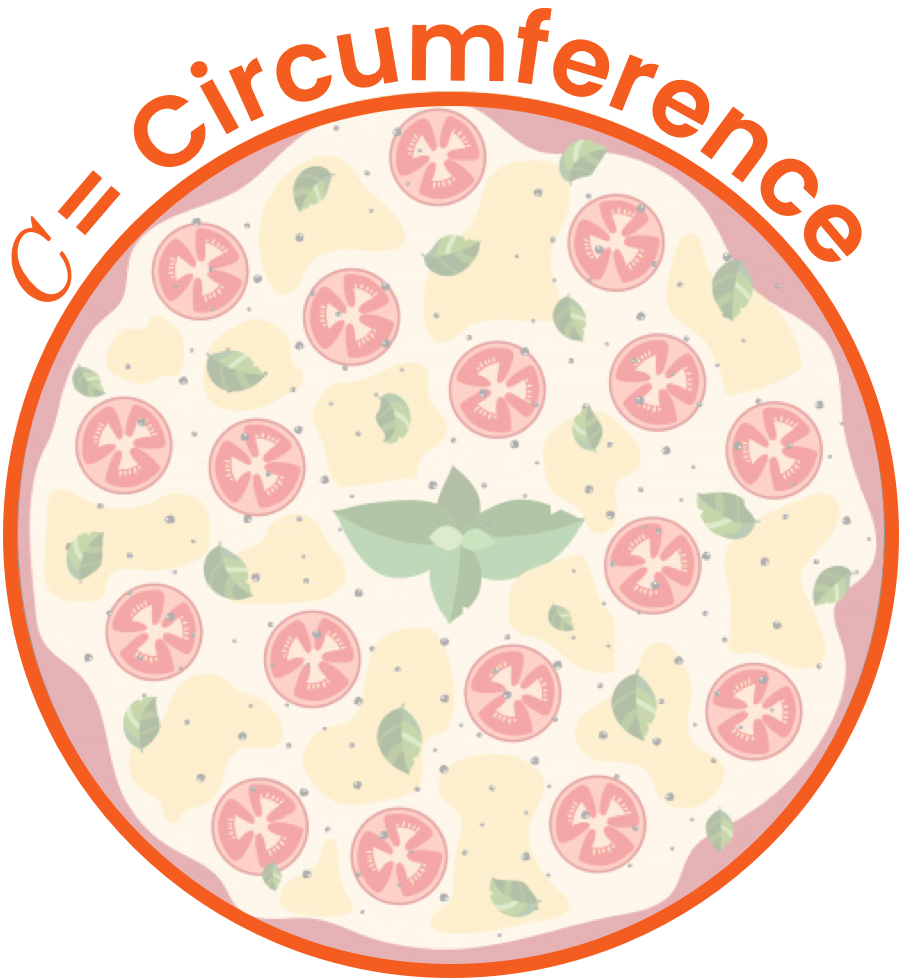KEY STEPS

## How to Find Circumference & Area of Circles

### Step 1. Identify the unknown.

What are you trying to solve for?
Since the unknown is the circumference, you need to solve for the distance around the circle, which is like the crust of a pizza.

### Step 2. Identify the given.

What information do you already have?

### Step 3. Select the correct formula(s).

You can use the following formula(s) to solve for the circumference the given the radius:

### Step 4. Plug in the given and solve for the unknown.

Plug in the given value into the formula and solve for the unknown using inverse operations as needed.
LESSON
Diameter Formula

## Diameter Formula

The diameter represents the width of a circle or, in pizza terms, the distance across a pizza.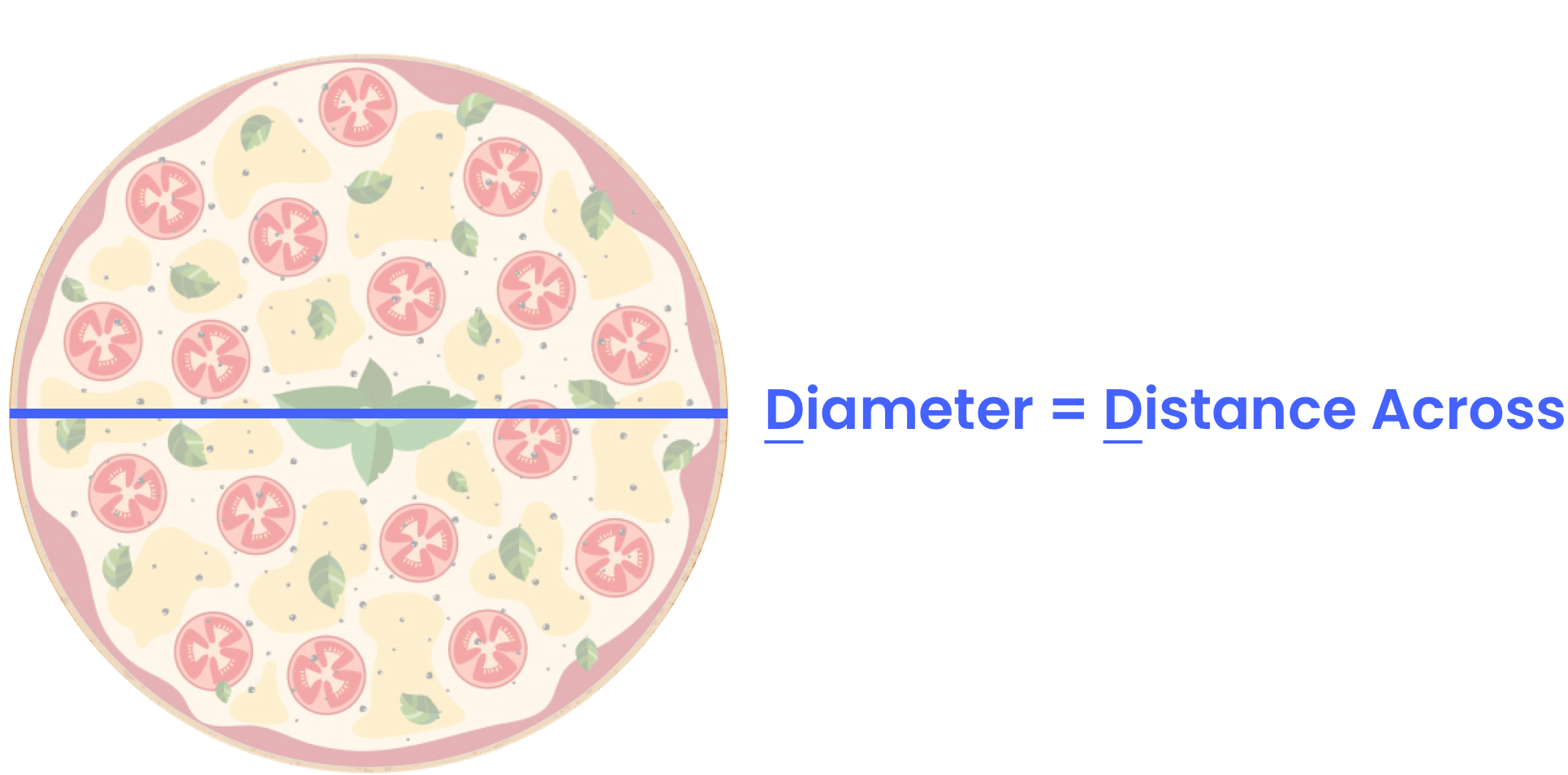If we divide the diameter in half, we get the radius. The radius represents half of the width of a circle, or in pizza terms, the length of a slice of pizza.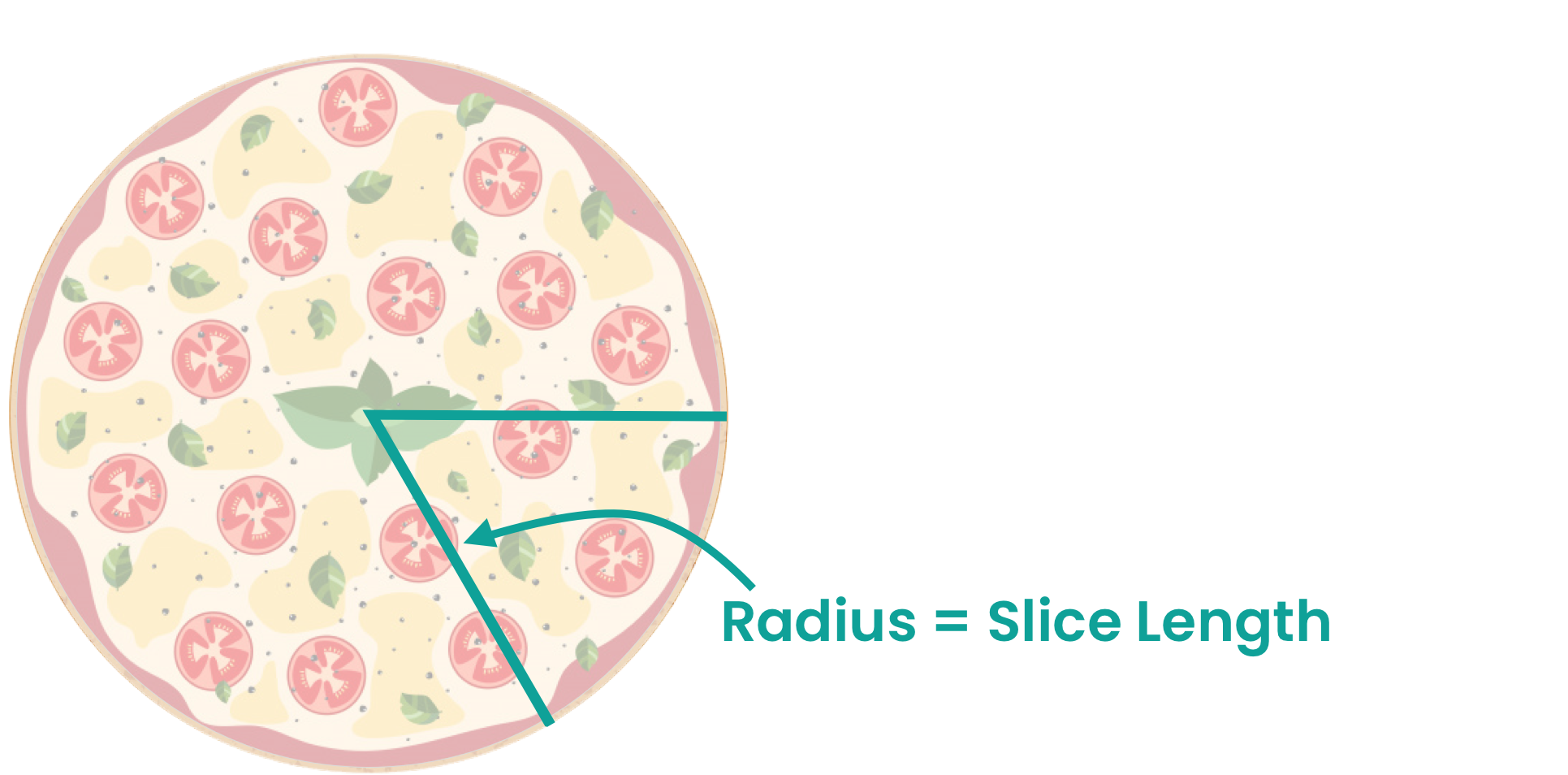The diameter and radius are very important because we need at least one of them to solve for the circumference and area of a circle.
If we’re given the radius, we can easily find the diameter: . If we’re given the diameter we can easily find the radius: .
Continue to see an example of each case!
PRACTICE
Diameter Formula

## Practice: Diameter Formula

Question 1 of 4: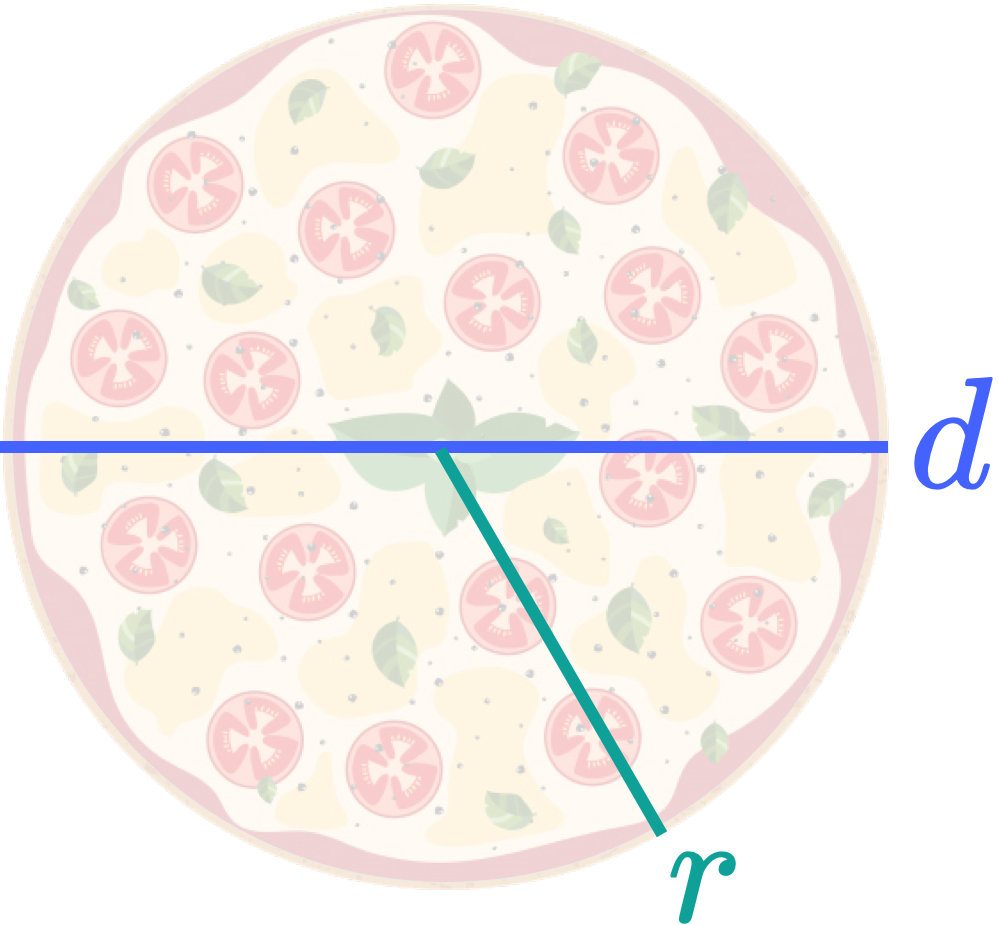### Step 1. Identify the unknown.

What are we trying to solve for?

LESSON
Circumference Formula

## Circumference Formula

The circumference represents the distance around a circle, or in pizza terms, the crust.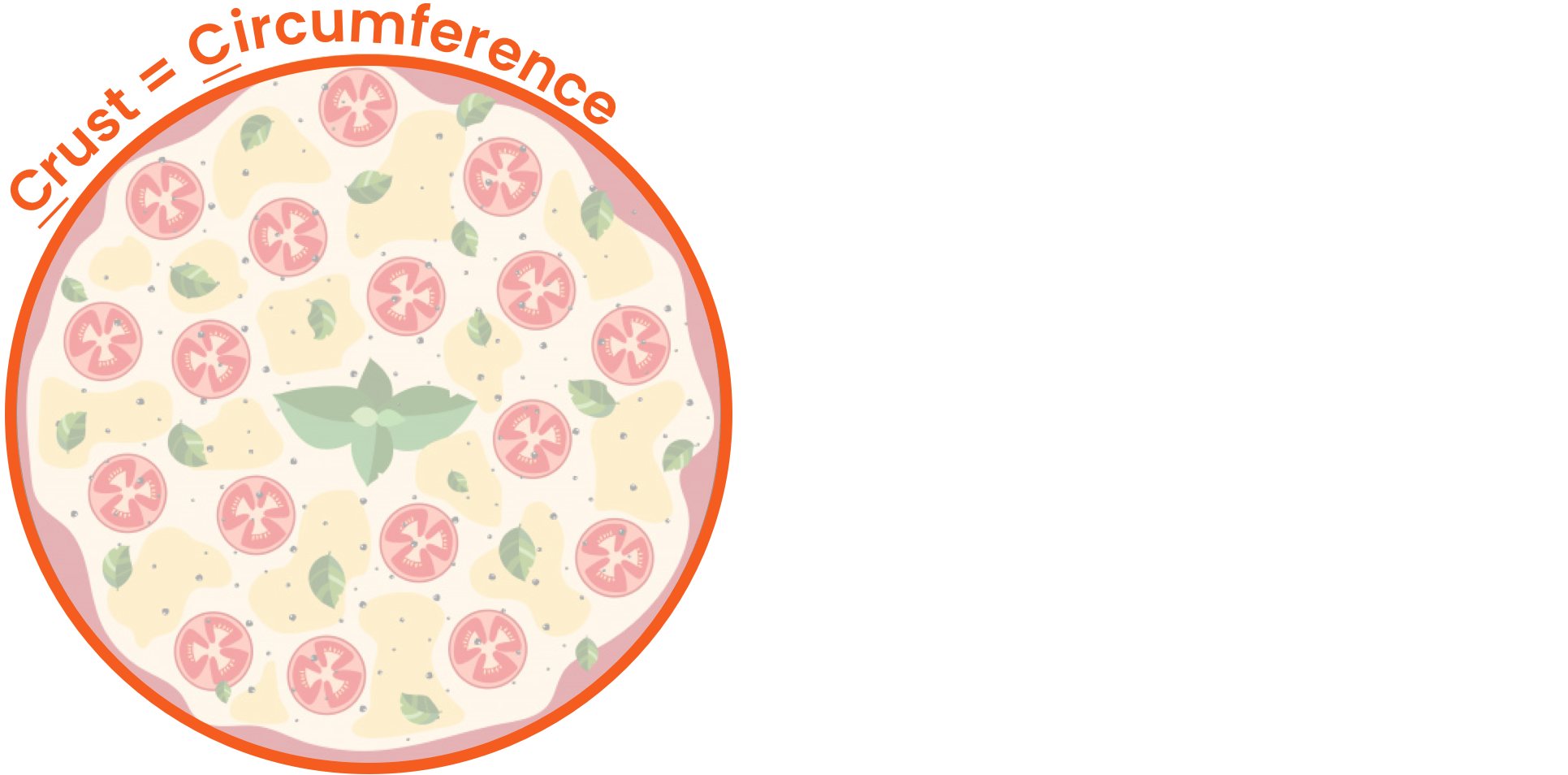Since the diameter is just radius, we can use either one to find the circumference of a circle.Continue below to walkthrough examples of how to find the circumference of a circle given the radius or diameter and vice versa!
PRACTICE
Circumference Formula

## Practice: Circumference Formula

Question 1 of 4: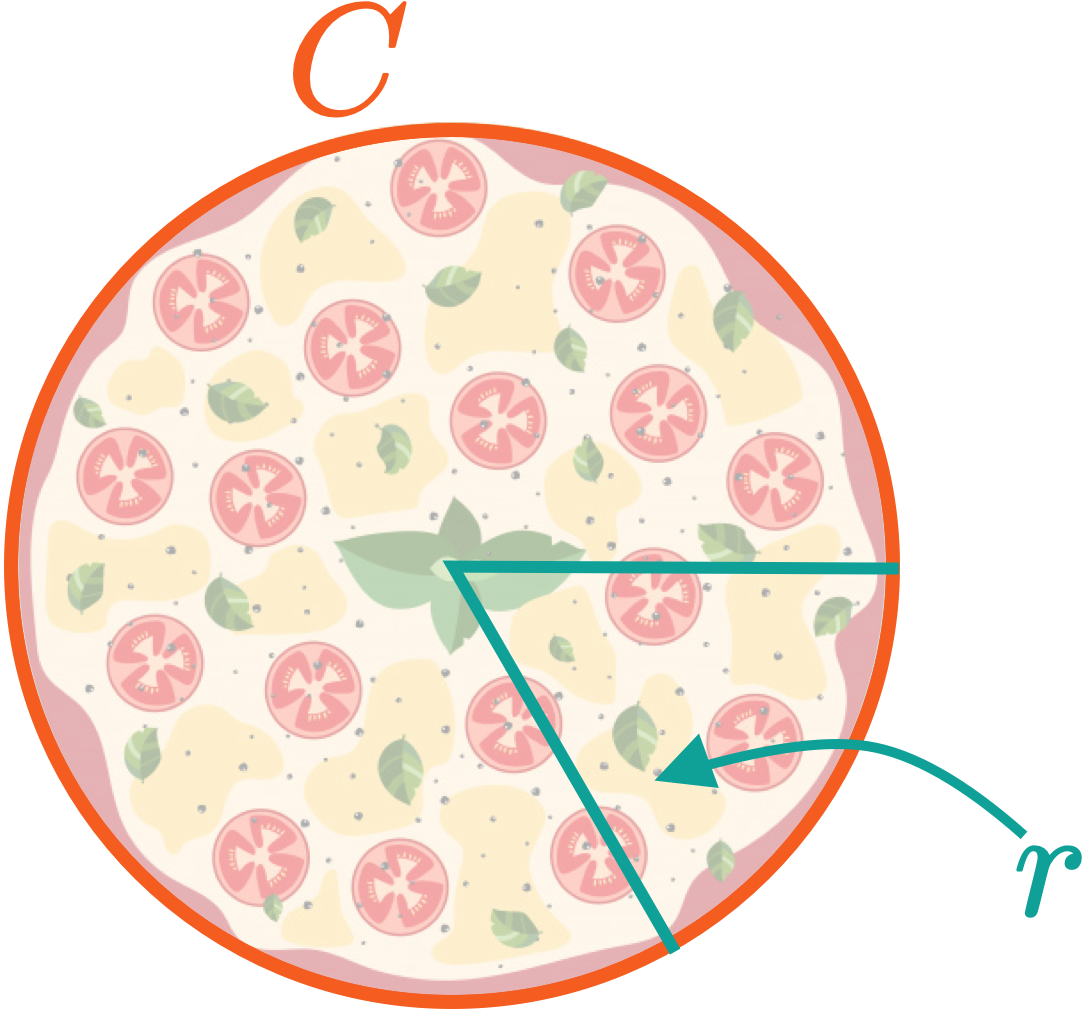### Step 1. Identify the unknown.

What are we trying to solve for?

LESSON
Area Formula

## Area Formula

The area represents the total amount of space inside a circle or, in pizza terms, the total amount of pizza.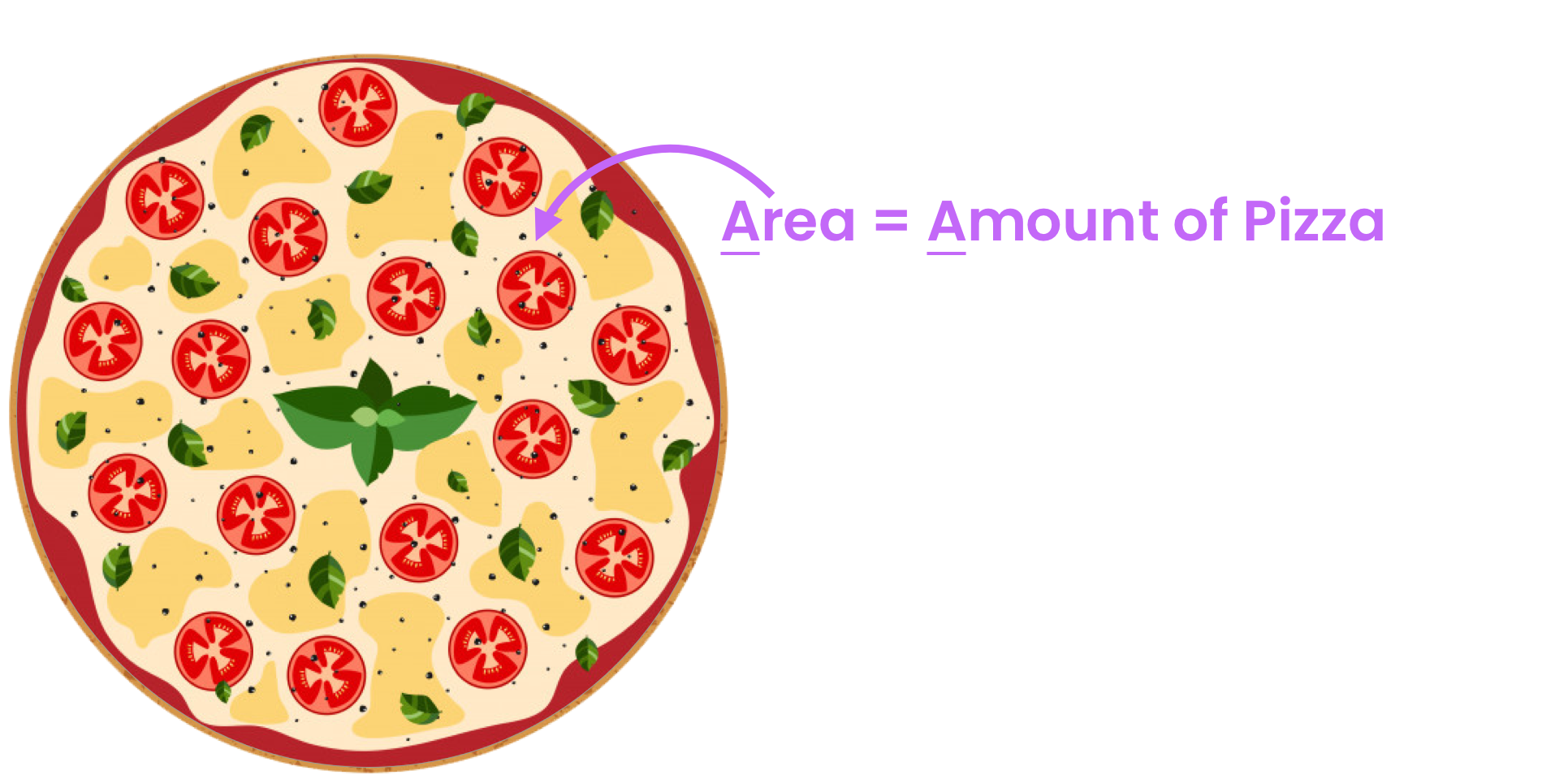The formula for the area of a circle is:If we’re given the radius, we can easily find the area.
If instead, we’re given the diameter, we first have to make a pit stop to find the radius using . Once we have the radius, we can plug it into the circle area formula and solve!
Continue below to walkthrough examples of how to find the area of a circle given the radius or diameter and vice versa!
PRACTICE
Area Formula

## Practice: Area Formula

Question 1 of 4: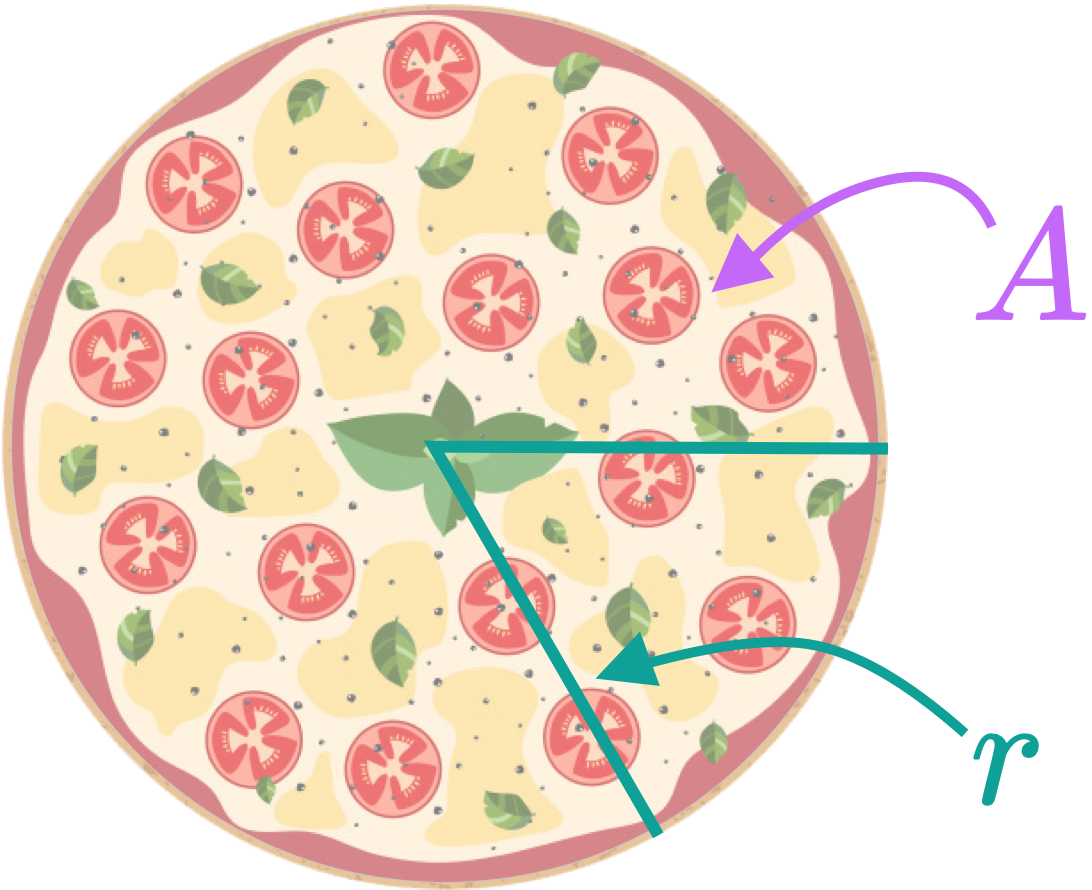### Step 1. Identify the unknown.

What are we trying to solve for?

CONCLUSION
Amazing work, look at you go! Thanks for checking out this lesson ☺️🙏. Where to next?Leave Feedback